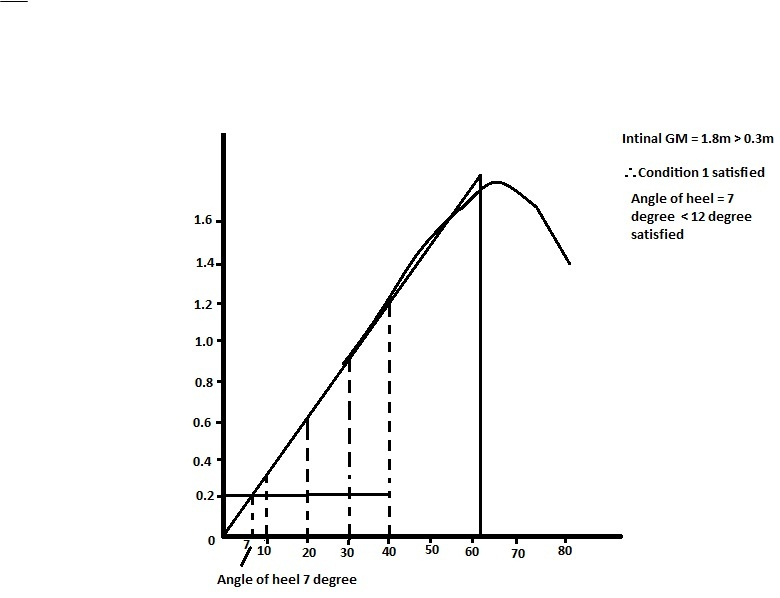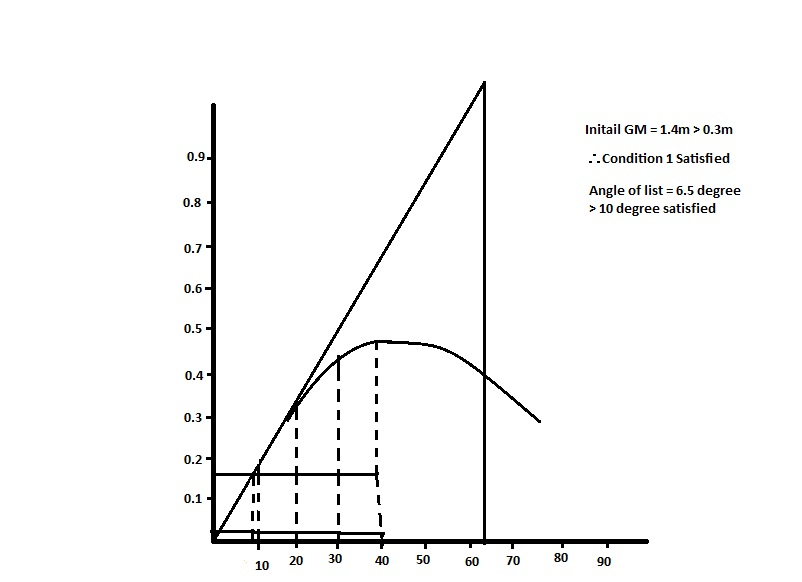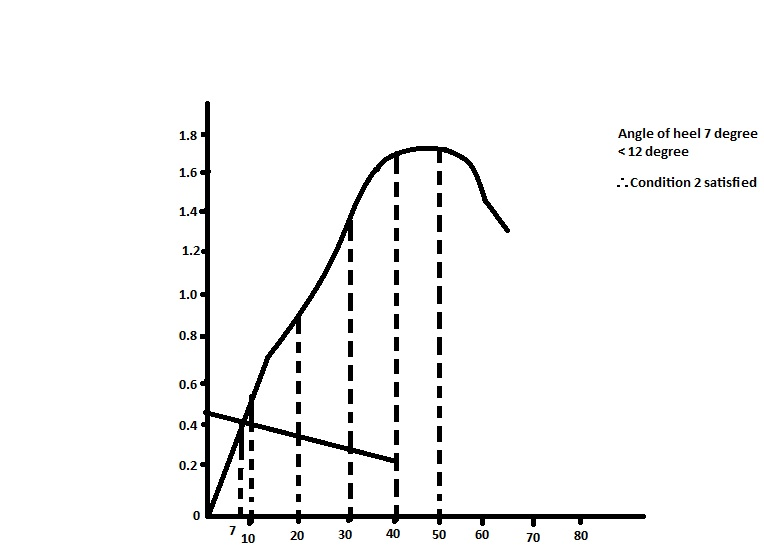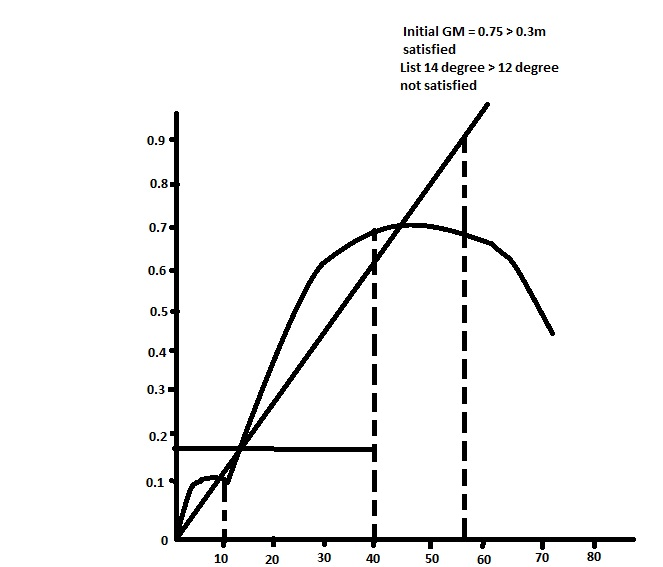C L DUBEY – EXERCISE – 11 (Grain Stability) |
Miscelleneous

# C L DUBEY – EXERCISE – 11 (Grain Stability)

###### Solution –

Given VHM = 3960m4
Weight heeling moment = 3960/1.2
= 3300m4
λ0 = 0.2m, λ40 = 0.16m

• GM = 0.776m

Therefore, condition 1 satisfied

• Angle of heel = 120 = Marginal.Heel Ordinate SM Product 0 0.0 1 0 10 0.15 4 0.60 20 0.33 2 0.66 30 0.645 4 2.28 40 0.610 1 0.610 4.45

Area = 10/3 ⨯ 4.45/57.3

Area of triangle = 0.5 ⨯ 12 ⨯ 0.19/57.3

Area of trapezium = 0.5 ⨯ (0.19 + 0.16) ⨯ 28/57.3

Residual area = 0.16m radian ˃ 0.075
Therefore, condition 3 satisfied.

###### Solution –

Given λ0 = 0.275, λ40 = 0.22Heel Ordinate SM Product 0 0 1 o 10 0.35 4 1.4 20 0.764 2 1.53 30 1.227 4 4.91 40 1.551 1 1.55 SOP = 9.30

Area of triangle = 0.5 ⨯ 7 ⨯ 0.265/57.3

Area of trapezium = 0.5 ⨯ (0.265 + 0.22) ⨯ 33/57.3

Therefore, condition 3 satisfied

###### Solution –

Given, λ0 = 0.18m, λ40 = 0.14mList Ordinate SM Product 0 0.0 1 0 10 0.263 4 1.052 20 0.472 2 0.944 30 0.604 4 2.416 40 0.631 1 0.631 SOP = 5.043

Area = 10/3 ⨯ 5.043/57.3

Area of triangle = 0.5 ⨯ 6.5 ⨯ 0.1735/57.3

Area of trapezium = 0.5 ⨯ (0.1735 + 0.14) ⨯ (4.0 – 6.5)/57.3

Residual area = 0.192m > 0.075m radian
Therefore, All condition satisfied.

###### Solution –

Given, KM = 11.30
KG = 7.809m

GM = (KM – KG)
= 3.491m > 0.3m

Therefore, Condition 1 satisfied

Now total volumetric heeling moment = 21321
Weight heeling moment = VHM/SF
= 16400.7692

Heeling arm = λ0 = 16400.76923/35186
= 0.466m
λ40 = 0.373m

 heel 5 12 15 30 45 60 KN 1 2042 2.9 5.6 7.3 8.05 KG SinӨ 0.68 1.623 2.021 3.904 5.522 6.763 GZ 0.32 0.797 0.879 1.696 1.778 1.287Heel Ordinate SM Product 0 0 1 0 10 0.65 4 2.6 20 1.05 2 2.10 30 1.696 4 6.784 40 1.760 1 1.760 SOP = 13.244

Area = 10/3 ⨯ 13.244/57.3

Area of triangle = 0.5 ⨯ 7 ⨯ 0.420/57.3

Area of trapezium = 0.228 m radian
Therefore, Residual area = 0.516 m radian > 0.075
Condition 3 satisfied.

###### Solution –

λ0 = 0.18, λ40 = 0.144List ordinate SM Product 0 0 1 0 10 0.135 4 0.54 20 0.472 2 0.944 30 0.604 4 2.416 40 0.631 1 0.631 4.531

Area = 10/3 ⨯ 4.531/57.3

Area of triangle = 0.5 ⨯ 17 ⨯ 0.162/57.3

Area of trapezium = 0.5 ⨯ (0.163 + 0.144) ⨯ 23/57.3

Therefore, Ship doesn’t comply.

###### Hence, vessel does not satisfy intact stability requirement for grain cargo or grain loading criteria.